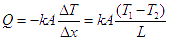# Heat transfer by conduction across a furnace wall

During heat transfer by conduction across a furnace wall, heat flows through a solid material, such as the walls of a furnace, from a region of higher temperature to a region of lower temperature. This transfer of heat occurs due to the collisions of particles within the solid material.

A furnace is a device in which heat is generated and transferred to materials with the object of bringing about physical and chemical changes. Calculating the heat transfer rate across a furnace wall is very important for designing and improving the efficiency of furnaces.

## Heat transfer - conduction

In a furnace, heat transfer through the furnace wall occurs primarily by conduction since the solid wall material is the primary barrier between the high-temperature furnace interior and the surrounding cooler environment. Heat transfer by conduction is the transfer of heat through a material without any net motion of the material itself. In other words, it occurs through a solid, liquid or gas when heat is transferred from a region of high temperature to a region of lower temperature by molecular collisions, without any movement of the material as a whole.

Conduction occurs when there is a temperature difference across a material, resulting in a transfer of energy from the hotter side to the colder side. The rate of heat transfer by conduction depends on the thermal conductivity of the material, the temperature gradient, and the cross-sectional area of the material.

Thermal conductivity is a measure of a material's ability to conduct heat. Materials with high thermal conductivity, such as metals, conduct heat more easily than materials with low thermal conductivity, such as ceramics.

The temperature gradient is the change in temperature per unit length of the material. The greater the temperature gradient, the faster the rate of heat transfer by conduction.

The cross-sectional area of the material also affects the rate of heat transfer by conduction. The larger the cross-sectional area, the greater the rate of heat transfer. The thickness of the furnace wall also affects the rate of heat transfer by conduction. Thicker walls offer greater resistance to heat transfer, slowing the rate of transfer.

## How to calculate rate of heat transfer across a furnace wall?

#### Problem Statement

Determine the rate of heat transfer by conduction per unit area, by means of conduction for a furnace wall made of fire clay. Furnace wall thickness is 6" or half a foot. Thermal conductivity of the furnace wall clay is 0.3 W/m·K. The furnace wall temperature can be taken to be same as furnace operating temperature which is 6500C and temperature of the outer wall of the furnace is 1500C.

#### Solution

Solution to this sample problem is quite straightforward as demonstrated below.For heat transfer by conduction across a flat wall, the heat transfer rate is expressed by following equation,For the given sample problem,

Temperature at inner wall of furnace = T1 = 6500C
Temperature at outer wall of furnace = T2 = 1500C
Thickness of furnace wall = L = 12" = 12 × 0.0254 m = 0.3048 m
Thermal conductivity of furnace wall material = k = 0.3 W/m·K

Hence, heat transfer rate per unit area of the wall is calculated as,

Q/A = k × (T1 - T2)/L
Q/A = 0.3×(650-150)/0.3048 W/m2 = 492.13 W/m2

This figure multiplied by the area of the furnace wall, will determine the total heat transfer rate in Joules/sec i.e. Watt.